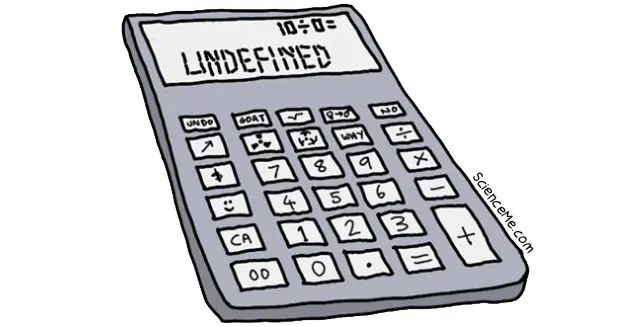# Why Can't You Divide by Zero?

### Enter any number into a calculator and divide by zero. What do you get? Undefined. What in the name of Turing's testicles does your calculator mean by undefined?

Undefined is not a real number. It is not even an irrational number. It is a mind-blowing abstraction that's not infinity, negative infinity, nor anywhere in between.

And yet, in the spirit of scientific enquiry, I'm going to try to illustrate it.Take a nice friendly number like 10.

What happens if you divide 10 by 10 ten? You get 1.

Let's mark this on our map.Stick with 10 and divide it by a smaller number, like 5.

How many 5s in 10? Just 2.Now rinse and repeat with smaller and smaller numbers:

10 / 5 = 2

10 / 2 = 5

10 / 1 = 10

10 / 0.5 = 20

10 / 0.1 = 100

10 / 0.001 = 10,000

10 / 0.000000001 = 10,000,000,000As you can see, the smaller the denominator, the bigger the result. In other words, as the denominator approaches zero, the result approaches infinity.

So if we were to actually divide 10 by zero, the result should be infinity, right?

Not so fast! Look at what mind-fizzing symmetry occurs if we approach zero from the other direction:

10 / -10 = -1

10 / -5 = -2

10 / -2 = -5

10 / -1 = -10

10 / -0.5 = -20

10 / -0.1 = -100

10 / -0.001 = -10,000

10 / -0.000000001 = -10,000,000,000See that? As the denominator approaches zero from the negative side, the result approaches negative infinity.This puts us in quite a pickle. The diminishing positive denominators suggest dividing by zero would give a result of infinity. And yet the diminishing negative denominators suggest the exact opposite: a result of negative infinity.

And that's why your calculator—a veritable pocket deity in all things mathematical—struggles to answer the seemingly simply sum of anything divided by zero.

Instead it gives you the disturbingly beautiful term: undefined.